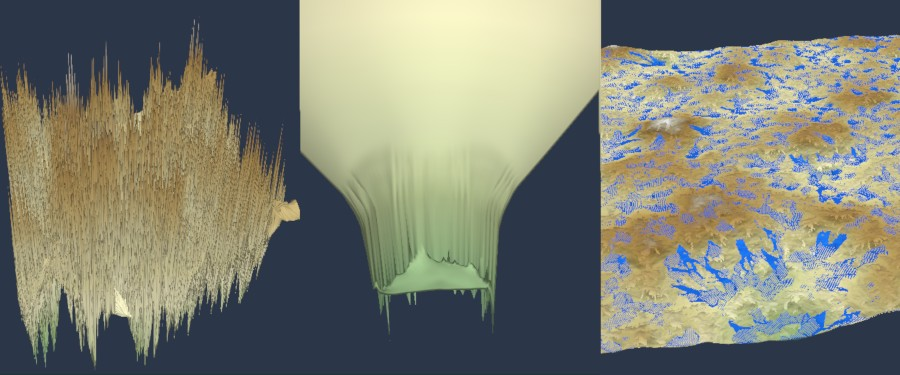### Identifying and avoiding digital instabilities

Some Instant Terra nodes produce a geometrically degenerate terrain. These geometric artifacts can appear as noise, oscillations, holes, aliasing, etc.To avoid these problems, we can:

• Increase the spatial resolution, i.e. the cell size.
• Decrease the time increment parameter (you can modify this parameter in some nodes).
• Simulate a scene where quantity variations are smaller, for example, decreasing the amount of rain, decreasing the force of erosion, or working on flatter terrain with fewer cliffs.

For information purposes, here are some details on the origin of oscillations:

A numerical simulation works by iterations, i.e. in steps. Each iteration is calculated using the results of the previous iteration. During a simulation, some data vary with each step of simulation (the amount of water, the terrain height, the temperature, etc.). Two successive iterations of a simulation correspond to two successive snapshots of the situation. It is possible to set by how long these two snapshots are separated. This is the notion of a time increment (also called simulation step or delta t).

Generally, we can assume that if we run a numerical simulation, all the iterations will take the same amount of time to compute (here, we refer to the time in seconds for the computer to compute the result of an iteration). If we want to simulate a physical phenomenon that lasts 1000 units of time, we can imagine simulating it either with (A) 1000 iterations with a time step of 1 or with (B) 2000 iterations with a time step of 0.5. The main difference between (A) and (B) lies in the total computation time for the user (B will take twice as long to compute); B will also be a little more accurate.

Intuition prompts the user to request (C): a simulation of 1 iteration of 1000 units of time. Unfortunately, this is where the numerical instabilities of the explicit models come into play. The calculations become false when the CFL condition  is no longer respected, i.e. when the spatial resolution is too small, the increment of time too large, or the variations in quantities too significant during the simulation.

Reference:  Courant-Friedrichs-Lewy condition